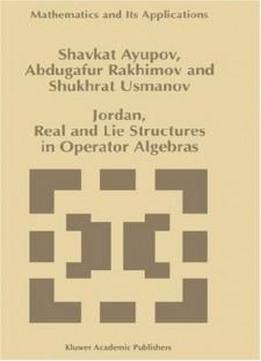# Jordan, Real And Lie Structures In Operator Algebras (mathematics And Its Applications) by Sh. Ayupov / 1997 / English / PDF

The theory of operator algebras acting on a Hilbert space was initiated in thirties by papers of Murray and von Neumann. In these papers they have studied the structure of algebras which later were called von Neu­ mann algebras or W* -algebras. They are weakly closed complex *-algebras of operators on a Hilbert space. At present the theory of von Neumann algebras is a deeply developed theory with various applications. In the framework of von Neumann algebras theory the study of fac­ tors (i.e. W* -algebras with trivial centres) is very important, since they are comparatively simple and investigation of general W* -algebras can be reduced to the case of factors. Therefore the theory of factors is one of the main tools in the structure theory of von Neumann algebras. In the middle of sixtieth Topping [To 1] and Stormer [S 2] have ini­ tiated the study of Jordan (non associative and real) analogues of von Neumann algebras - so called JW-algebras, i.e. real linear spaces of self­ adjoint opera.tors on a complex Hilbert space, which contain the identity operator 1. closed with respect to the Jordan (i.e. symmetrised) product INTRODUCTION 2 x 0 y = ~(Xy + yx) and closed in the weak operator topology. The structure of these algebras has happened to be close to the struc­ ture of von Neumann algebras and it was possible to apply ideas and meth­ ods similar to von Neumann algebras theory in the study of JW-algebras.

The theory of operator algebras acting on a Hilbert space was initiated in thirties by papers of Murray and von Neumann. In these papers they have studied the structure of algebras which later were called von Neu­ mann algebras or W* -algebras. They are weakly closed complex *-algebras of operators on a Hilbert space. At present the theory of von Neumann algebras is a deeply developed theory with various applications. In the framework of von Neumann algebras theory the study of fac­ tors (i.e. W* -algebras with trivial centres) is very important, since they are comparatively simple and investigation of general W* -algebras can be reduced to the case of factors. Therefore the theory of factors is one of the main tools in the structure theory of von Neumann algebras. In the middle of sixtieth Topping [To 1] and Stormer [S 2] have ini­ tiated the study of Jordan (non associative and real) analogues of von Neumann algebras - so called JW-algebras, i.e. real linear spaces of self­ adjoint opera.tors on a complex Hilbert space, which contain the identity operator 1. closed with respect to the Jordan (i.e. symmetrised) product INTRODUCTION 2 x 0 y = ~(Xy + yx) and closed in the weak operator topology. The structure of these algebras has happened to be close to the struc­ ture of von Neumann algebras and it was possible to apply ideas and meth­ ods similar to von Neumann algebras theory in the study of JW-algebras.

views: 572